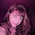## Wednesday, May 14, 2014

### Plotting bars at the tips of a tree, part II

Earlier today, I responded to an R-sig-phylo request to be able to plot bars showing phenotypic trait values for species at the times of a circular or 'fan' tree. I have now added this function (plotTree.wBars) to the phytools package. It can be downloaded & installed from source here.

In addition to plotting a circular tree, this function version also:

(1) Plots a square phylogram in "rightward" or "leftward" orientation.

(2) Plots a stochastic character mapped tree (using plotSimmap instead of plotTree internally).

(3) Can accept most of the arguments of plotTree & plotSimmap (see the documentation page for plotSimmap for more information.)

Here's a demo:

> packageVersion("phytools")
 ‘0.4.8’
> tree<-pbtree(n=50)
> x<-fastBM(tree,bounds=c(0,Inf))
> plotTree.wBars(tree,x)
> Q<-matrix(c(-1,1,1,-1),2,2)
> tree<-sim.history(tree,Q)
> plotTree.wBars(tree,x,method="plotSimmap", colors=setNames(c("blue","red"),1:2),lwd=3)
> plotTree.wBars(tree,x,type="fan",method="plotSimmap", colors=setNames(c("blue","red"),1:2),lwd=3,scale=0.5, width=0.2)

That's it.

#### 1 comment:

1.Hi Liam,

great tool. I am just wondering how to assign a meaningful variable to x, as opposed to a simulated variable?